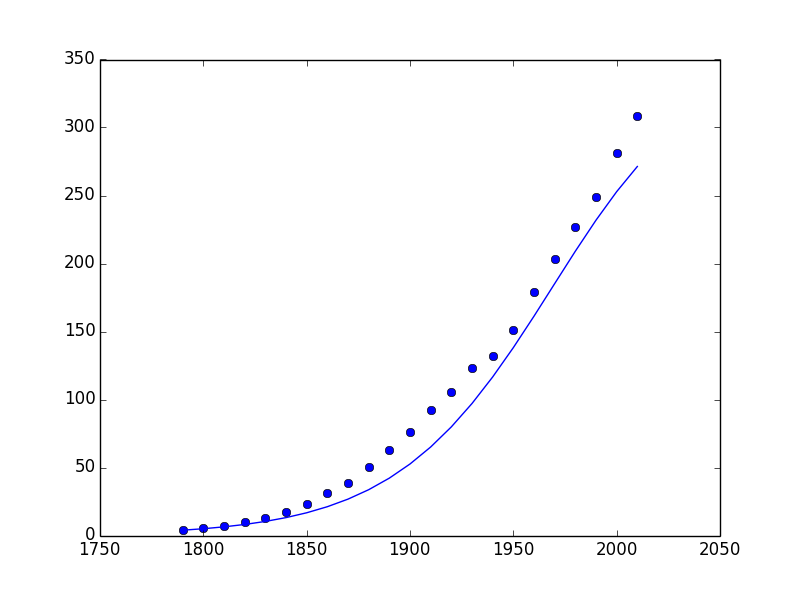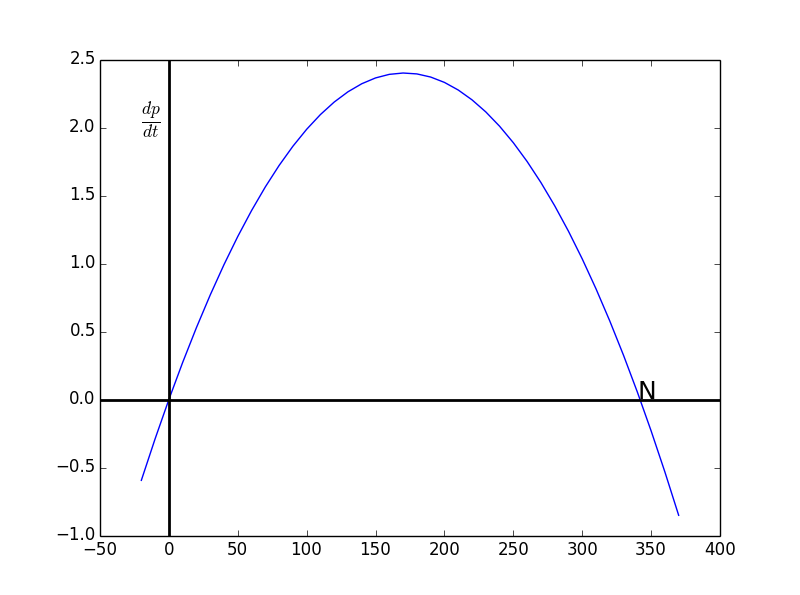通过微分方程建模

1 数学建模（Mathematical Modeling）

1. 提出前提假设（assumption）
2. 识别相关的变量参数
3. 根据1中前提假设对2中的变量构建数学公式

1. 自变量（independent variable）
2. 因变量（dependent variable）
3. 参数（parameter）

1. 前提假设：跳伞者只收到重力和摩擦力影响，摩擦力与速度的平方成正比，以及牛顿第二定律：
$F=ma$
2. 变量：
• 自变量：t 时间（秒）
• 因变量：v 速度
• 参数：
1. g 重力常数
2. m 跳伞者的质量
3. k 阻力的系数
3. 建模：
• 等式左边，跳伞者收到力：
$F=mg-kv^2$
• 等式右边，质量乘以加速度：
$mfrac{dv}{dt}$
• 模型为：
$mg-kv^2=mfrac{dv}{dt}$

$mg-kv^2=mfrac{dv}{dt}$

2 建模人口增长1. 前提假设： 人口的增长速率与人口数量成正比
2. 变量：
• 自变量: t 时间（年，从1790年开始）
• 因变量：p 人口 （百万）
• 参数：
1. k 人口的增长速率与人口数量所成的比率
3. 模型：
• 等式左边，人口的增长率：
$frac{dp}{dt}$
• 等式右边：
$kp$
• 模型为：
$frac{dp}{dt}=kp$

$frac{dp}{dt}=kp$
$frac{1}{p}frac{dp}{dt}=k$
$intfrac{1}{p}frac{dp}{dt}dt=int{k}dt$
$intfrac{1}{p}dp=kt+C$
$ln(p)=kt+C$
$p=e^{kt+C}$
$p=e^{kt}e^C$
$p=Ke^{kt}$
$p(t)=Ke^{kt}$

$p(0)=K=3.9$
$p(10)=3.9e^{10k}=5.3implies k = 0.03067$

$p(t)=3.9e^{0.03067t}$3 有限资源与罗吉斯特人口模型

• 人口数量较少时，人口的增长速率与人口数量成正比
• 随着人口的增长，人口的相对增长率（relative growth rate）逐渐减小
人口的相对增长率定义为：
$text{relative growth rate} = frac{(frac{dp}{dt})}{p}=frac{1}{p}frac{dp}{dt}$

1. 前提假设：人口的增长率与人口数量呈反比，即人口的相对增长率与人口数量成反比
2. 变量：
• 自变量: t 时间（年，从1790年开始）
• 因变量：p 人口 （百万）
• 参数：
1. k
2. m 人口增长速度随人口数量变化而变化的比率
3. 模型：
• 等式左边，人口的相对增长率：
$frac{1}{p}frac{dp}{dt}$
• 等式右边：
$k+mp$
• 模型为：
$frac{1}{p}frac{dp}{dt}=k+mp$

t p
0 3.9
10 5.3
20 7.2

$frac{dp}{dt}bigg|_{t=10}approxfrac{p(20)-p(0)}{20}= 0.165$$frac{1}{p}frac{dp}{dt}=0.02808 + 0.000082111times p$

$frac{dp}{dt}=0.02808p+0.000082111times p^2$4 定性分析（Qualitative analysis）

3中得到人口的相对增长率，$frac{1}{p}frac{dp}{dt}=k+mp$，从函数图上看，回归线$k+mp$$p$轴于点$N$

• 在人口$p时，增长率是正的，表示人口将增加
• 在人口$p>N$时，增长率是负的，表示人口将减少

$N$$p$表示$m$并带入罗吉斯特模型获得：
$frac{1}{p}frac{dp}{dt}=k-frac{k}{N}p$

$frac{dp}{dt}=kp-frac{k}{N}p^2$1. $p(0)=0implies forall t > 0, p(t) = 0$
2. $p(0)=Nimplies forall t >0, p(t) = N$
3. $0 < p(0) < N implies lim_{trightarrow infty}p(t) = N$
4. $p(0)>N implies lim_{trightarrow infty}p(t) = N$